Home Practice
For learners and parents For teachers and schools
Textbooks
Full catalogue
Pricing SupportLog in

We think you are located in United States. Is this correct?

# Equivalent forms of descriptions of relationships

## 21.2 Equivalent forms of descriptions of relationships

In Chapter 5, you already learnt how to complete tables using equations and how to read flow diagrams to analyse different functions and relationships. We will now look at more examples of using an equation to complete a table of values. Remember, the table of values can help you to sketch graphs and look for patterns in a relationship.

When working with integers, pay a special attention to operations with negative numbers. For example, when you need to substitute $$x = - 10$$ into the equation $$y = x^{2}$$, make sure you use brackets around the input value:

\begin{align} y &= ( - 10)^{2} \\ &= 100 \end{align}

If the relationship has fractions and integers, look out for any sign change after substituting a negative number in the numerator or denominator. For example, if $$x = - 2$$ and $$y = - \frac{2x}{6}$$, then

\begin{align} y &= - \frac{2( - 2)}{6} \\ &= - \frac{- 4}{6} \\ &= - \left( - \frac{2}{3} \right) \\ &= + \frac{2}{3} \\ &= \frac{2}{3} \end{align}

## Worked Example 21.1: Using equations to complete tables

Fill in the table of values below for the equation, $$y = - \frac{x}{2} - 1$$.

 $$x$$ $$−6$$ $$−4$$ $$−2$$ $$−1$$ $$1$$ $$6$$ $$y$$ $$2$$ $$1$$ $$0$$ $$−4$$

### Use the equation to find the first missing number.

The input for the first missing number is $$x = - 1$$. Substitute this value into the given equation:

\begin{align} y &= - \frac{x}{2} - 1 \\ y &= - \frac{(- 1)}{2} - 1 \\ y &= + \frac{1}{2} - 1 \\ y &= - \frac{1}{2} \end{align}

So, the first missing value is $$- \frac{1}{2}$$.

### Use the equation to find the second missing number.

The input for the second missing number is $$x = 1$$. Substitute this value into the given equation:

\begin{align} y &= - \frac{x}{2} - 1 \\ y &= - \frac{1}{2} - 1 \\ y &= - 1\frac{1}{2} \end{align}

So, the second missing value is $$- 1\frac{1}{2}$$.

All the pairs $$(x; y)$$ of corresponding $$x$$ and $$y$$ values are called the solutions of the given equation. This is because when one value is substituted into the equation, it holds true for the second value in the same pair.

temp text

Some formulas have more than two variables. When there are three variables, we need to find a “triplet” that satisfies the relationship.

## Worked Example 21.2: Using formulas with more than two variables

Consider this equation:

$p = \frac{3m}{4} - \frac{n}{4} + 9$

Solutions to this equation are the ordered triplets: $$(m;n;p)$$.

Use the equation to determine the final value in the following ordered triplet so that it solves the equation: $$( - 8;12;p)$$.

### Work out the input values.

The equation has three variables in it. So, you must work out the values of $$m$$ and $$n$$ which you should use to get the value of $$p$$ in the ordered triplet.

The question states that “solutions to this equation are ordered triplets: $$(m;n;p)$$”. This shows you how the triplets are organised: the variable $$m$$ comes first, followed by $$n$$. Therefore, in the triplet $$( - 8;12;p)$$ the $$−8$$ must be the value of $$m$$ and $$12$$ must be the value of $$n$$.

The input values from the question are $$m = - 8$$ and $$n = 12$$.

### Evaluate the equation to get the answer for $$p$$.

Once you have the input values, just substitute them into the equation and work out the output value, $$p$$.

\begin{align} p &= \frac{3m}{4} - \frac{n}{4} + 9 \\ p &= \frac{3( - 8)}{4} - \frac{12}{4} + 9 \\ &= \frac{- 24}{4} - \frac{12}{4} + 9 \\ &= - 6 - 3 + 9 \\ &= - 9 + 9 \\ &= 0 \end{align}

So, the answer is $$p = 0$$.

The ordered triplet we were looking for is $$( - 8;12;0)$$.

temp text

### Using relationships between variables to solve real-world problems

Functions and relationships between variables help us describe and solve different real-world problems. For example, the rule for converting temperature given in degrees Celsius to degrees Fahrenheit is given as: “Multiply the degrees Celsius by $$\text{1,8}$$ and then add $$32$$.”

We can use this rule to convert between different temperature units.

• $$0^{\circ}\text{C} \ \mathbf{\times \ \text{1,8} + 32} = 32^{\circ}\text{F}$$
• $$5^{\circ}\text{C} \ \mathbf{\times \ \text{1,8} + 32} = 41^{\circ}\text{F}$$
• $$20^{\circ}\text{C} \ \mathbf{\times \ \text{1,8} + 32} = 68^{\circ}\text{F}$$
• $$32^{\circ}\text{C} \ \mathbf{\times \ \text{1,8} + 32} = \text{89,6}^{\circ}\text{F}$$
• $$100^{\circ}\text{C} \ \mathbf{\times \ \text{1,8} + 32} = 212^{\circ}\text{F}$$

We can complete a table of values for this relationship.

 Temperature in degrees Celsius, $$^{\circ}\text{C}$$ $$0$$ $$5$$ $$20$$ $$32$$ $$100$$ Temperature in degrees Fahrenheit, $$^{\circ}\text{F}$$ $$32$$ $$41$$ $$68$$ $$\text{89,6}$$ $$212$$

We can then complete a flow diagram to represent this information.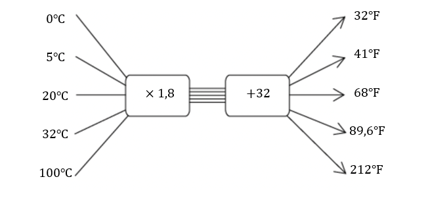We can also show this relationship as a graph, where the Celsius values are shown on the $$x$$-axis and the Fahrenheit values are shown on the $$y$$-axis. Plot the points and join them using a line.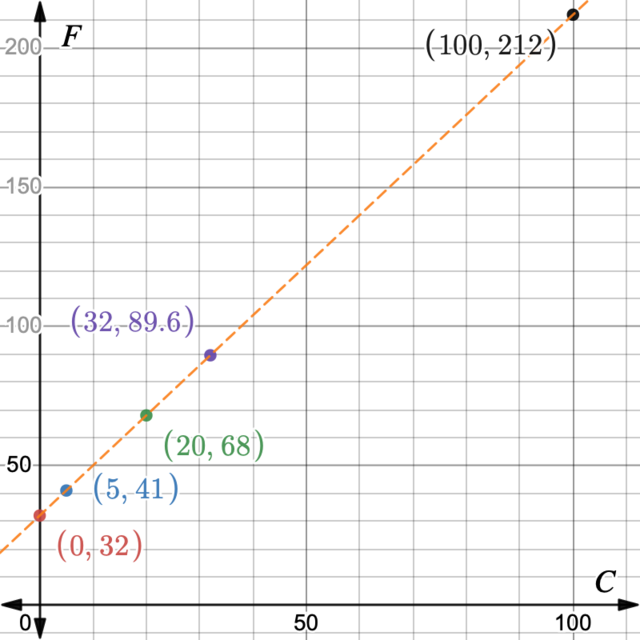This sometimes makes it easier to estimate the values without calculating them. For example, looking at the graph we can see that $$50^{\circ}\text{C}$$ must be approximately equal to $$122^{\circ}\text{F}$$.

Check this by calculating:

\begin{align} 50^{\circ}\text{C} \times \text{1,8} + 32 &= 90 + 32 \\ &= 122^{\circ}\text{F} \end{align}

The formula for temperature conversion can be written like this:

$$^{\circ}\text{F}=$$ $$^{\circ}\text{C} \times \text{1,8} + 32$$

## Worked Example 21.3: Use temperature conversion formula

Complete the table of values to convert the temperature readings between degrees Celsius to temperature units called kelvins. Show this relationship using a flow diagram and a graph.

 Temperature in degrees Celsius, $$^{\circ}\text{C}$$ $$0$$ $$20$$ $$32$$ Temperature in kelvins, K $$\text{283,25}$$ $$600$$

Here’s the formula to convert kelvins to degrees Celsius:

$^{\circ}\text{C} = K - \text{273,15}$

where $$^{\circ}\text{C}$$ is the unit temperature in degrees Celsius, and $$K$$ is the unit temperature in kelvins.

The formula to convert degrees Celsius to kelvins is the opposite:

$$K=$$ $$^{\circ}\text{C} + \text{273,15}$$

### Complete the missing measurements to find the temperature in degrees Celsius.

Use the formula $$^{\circ}\text{C} = K - \text{273,15}$$.

 Temperature in degrees Celsius, $$^{\circ}\text{C}$$ $$0$$ $$\text{10,10}$$ $$20$$ $$32$$ $$\text{326,85}$$ Temperature in kelvins, K $$\text{283,25}$$ $$600$$
\begin{align} ^{\circ}\text{C} &= \text{283,25} - \text{273,15} \\ &= \text{10,10} \end{align} \begin{align} ^{\circ}\text{C} &= 600 - \text{273,15} \\ &= \text{326,85} \end{align}

### Complete the missing measurements to find the temperature in Kelvin.

Use the formula $$K = ^{\circ}\text{C} + \text{273,15}$$.

 Temperature in degrees Celsius, $$^{\circ}\text{C}$$ $$0$$ $$\text{10,10}$$ $$20$$ $$32$$ $$\text{326,85}$$ Temperature in kelvins, K $$\text{273,15}$$ $$\text{283,25}$$ $$\text{293,15}$$ $$\text{305,15}$$ $$\text{600}$$
\begin{align} K &= 0 + \text{273,15} \\ &= \text{273,15} \end{align} \begin{align} K &= 20 + \text{273,15} \\ &= \text{293,15} \end{align} \begin{align} K &= 32 + \text{273,15} \\ &= \text{305,15} \end{align}

### Illustrate these temperature readings on the flow diagram.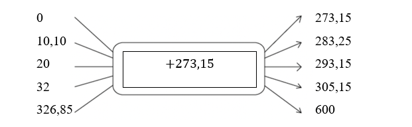### Plot the points to show the conversion graph.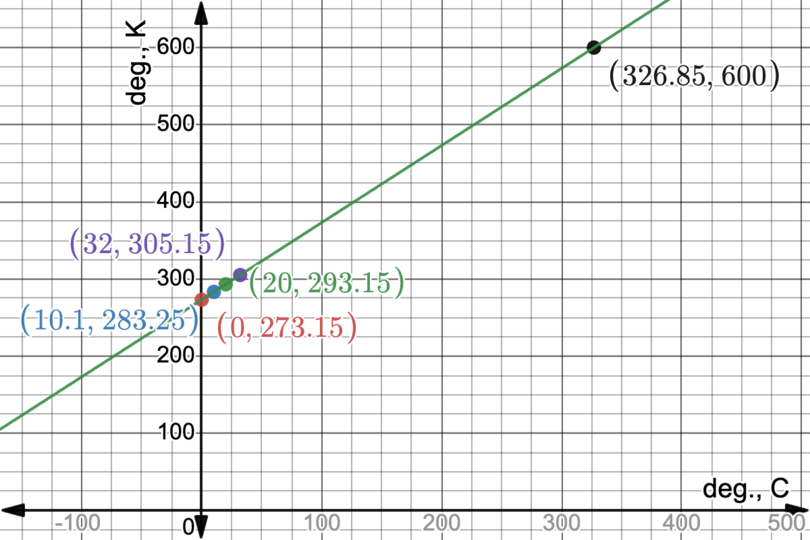Exercise 21.1

Another rule for converting temperature given in degrees Celsius to degrees Fahrenheit is given as: “Multiply the degrees Celsius by $$9$$, then divide the answer by $$5$$ and then add $$32$$ to the answer.”

1. Complete the flow diagram below.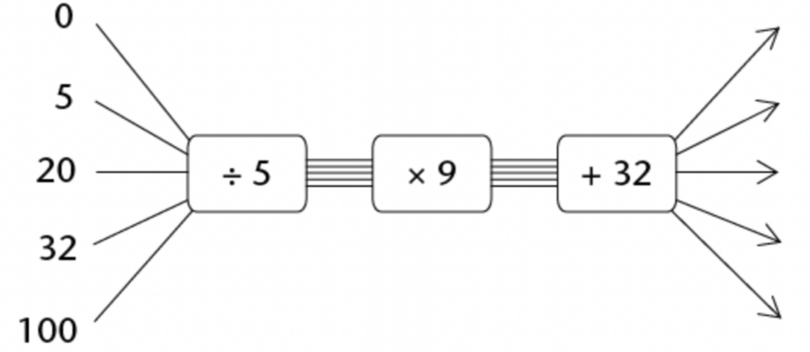2. Will this flow diagram below give the same output values as the flow diagram in question 1a)? Explain.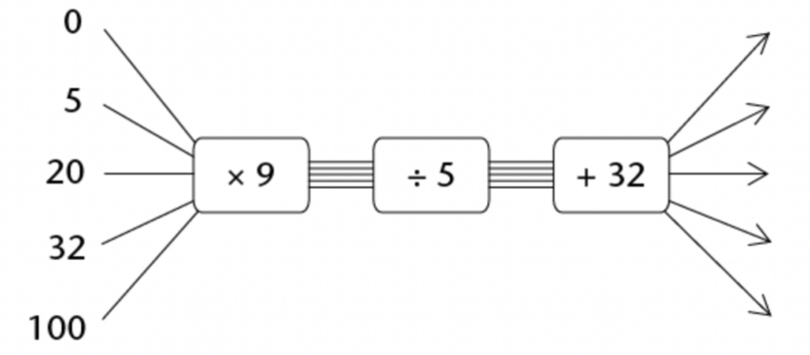3. Look at the flow diagram we used earlier, which is shown again below. Why do you think this flow diagram and the flow diagram given question 1a) will produce the same output numbers for the same input numbers, even though they have different operators?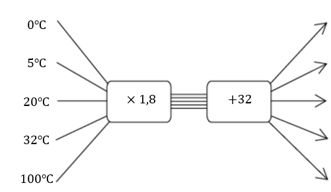1.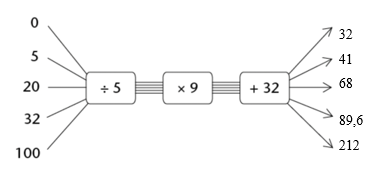2. The output values will be the same as in the given example, because $$( \div 5 \times 9)$$ is the same as $$( \times 9 \div 5)$$
3. The output values will be the same as in the given example, because $$( \div 5 \times 9)$$ is the same as $$\left( \times \frac{9}{5} \right) = ( \times \text{1,8})$$.

The rule for calculating the area of a square is: “Multiply the length of a side of the square by itself”.

1. Complete the table below.

 Length of side $$4$$ $$6$$ $$10$$ Area of square $$64$$ $$144$$
2. Complete a flow diagram to represent the information in the table.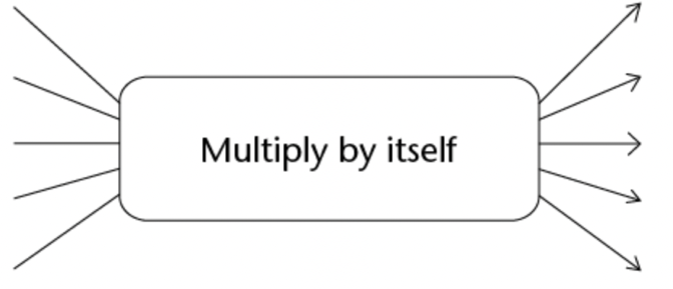1.  Length of side $$4$$ $$6$$ $$8$$ $$10$$ $$12$$ Area of square $$16$$ $$36$$ $$64$$ $$100$$ $$144$$
2.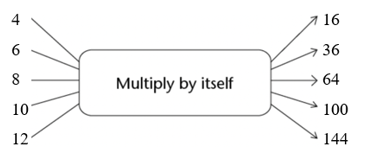The pattern below shows stacks of building blocks. The number of blocks in each stack is dependent on the number of the stack.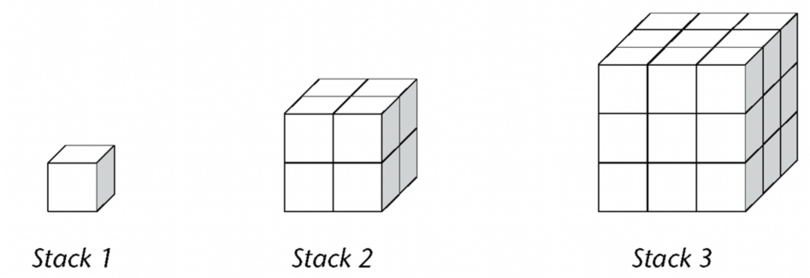1. Complete the table below to represent the relationship between the number of blocks and the number of the stack.
 Stack number $$1$$ $$2$$ $$3$$ $$4$$ $$5$$ $$6$$ $$7$$ $$8$$ Number of blocks $$1$$ $$8$$
1. Describe in words how the output values can be calculated.
2. Write a formula to match the description in b).
1.  Stack number $$1$$ $$2$$ $$3$$ $$4$$ $$5$$ $$6$$ $$7$$ $$8$$ Number of blocks $$1$$ $$8$$ $$27$$ $$64$$ $$125$$ $$216$$ $$343$$ $$512$$
2. “output = input cubed”
3. $y = x^{3}$